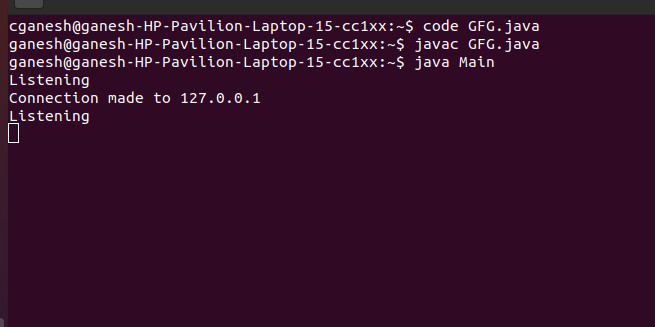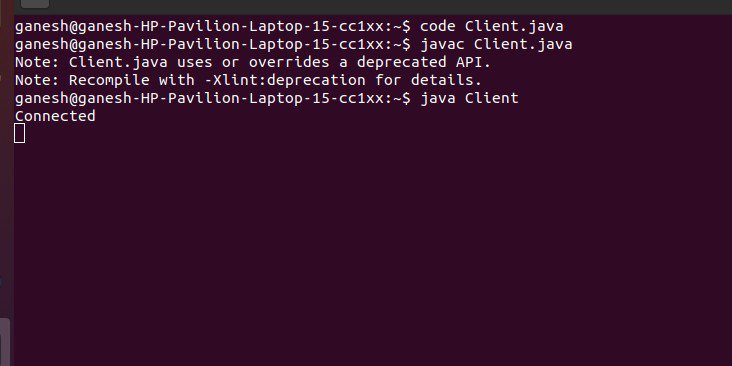# How to Make a Server to Allow the Connection to the Socket 6123 in Java?

• Last Updated : 11 Nov, 2020

A socket connection means the two machines have information about each other’s network location (IP Address) and TCP port. The java.net.Socket class represents a Socket. Here, we are going to look at the approach of connecting to socket 6123.

Approach:

• Create an object of Socket class and pass 6123 as an argument.
• Accept connections with accept() method of ServerSocket class.
• Get the address of the connection with getInetAddress() method of Socket class and getHostAddress() of InetAddress class.

Server Code:

## Java

 `// Make a server to allow the connection to the socket 6123`` ` `import` `java.io.IOException;``import` `java.net.InetAddress;``import` `java.net.ServerSocket;``import` `java.net.Socket;`` ` `public` `class` `Server {``    ``public` `static` `void` `main(String[] args) { check(); }``    ``private` `static` `void` `check()``    ``{``        ``try` `{``            ``// Creating object of ServerSocket class``            ``ServerSocket connection``                ``= ``new` `ServerSocket(``6123``);``           ` `            ``while` `(``true``) {``                ``System.out.println(``"Listening"``);`` ` `                ``// Creating object of Socket class``                ``Socket socket = connection.accept();`` ` `                ``// Creating object of InetAddress class``                ``InetAddress address``                    ``= socket.getInetAddress();``               ` `                ``System.out.println(``                    ``"Connection made to "``                    ``+ address.getHostAddress());``                ``pause(``1000``);``                   ` `                  ``// close the socket``                ``socket.close();``            ``}``        ``}``        ``catch` `(IOException e) {``            ``System.out.println(``"Exception detected: "` `+ e);``        ``}``    ``}``    ``private` `static` `void` `pause(``int` `ms)``    ``{``        ``try` `{``            ``Thread.sleep(ms);``        ``}``        ``catch` `(InterruptedException e) {``        ``}``    ``}``}`

OutputClient Code:

## Java

 `// A Java program for a Client`` ` `import` `java.net.*;``import` `java.io.*;`` ` `public` `class` `Client {``    ``// initialize socket and input output streams``    ``private` `Socket socket = ``null``;``    ``private` `DataInputStream input = ``null``;``    ``private` `DataOutputStream out = ``null``;`` ` `    ``// constructor to put ip address and port``    ``public` `Client(String address, ``int` `port)``    ``{``        ``// establish a connection``        ``try` `{``            ``socket = ``new` `Socket(address, port);``            ``System.out.println(``"Connected"``);`` ` `            ``// takes input from terminal``            ``input = ``new` `DataInputStream(System.in);`` ` `            ``// sends output to the socket``            ``out = ``new` `DataOutputStream(``                ``socket.getOutputStream());``        ``}``        ``catch` `(UnknownHostException u) {``            ``System.out.println(u);``        ``}``        ``catch` `(IOException i) {``            ``System.out.println(i);``        ``}`` ` `        ``// string to read message from input``        ``String line = ``""``;`` ` `        ``// keep reading until "Over" is input``        ``while` `(!line.equals(``"Over"``)) {``            ``try` `{``                ``line = input.readLine();``                ``out.writeUTF(line);``            ``}``            ``catch` `(IOException i) {``                ``System.out.println(i);``            ``}``        ``}`` ` `        ``// close the connection``        ``try` `{``            ``input.close();``            ``out.close();``            ``socket.close();``        ``}``        ``catch` `(IOException i) {``            ``System.out.println(i);``        ``}``    ``}`` ` `    ``public` `static` `void` `main(String args[])``    ``{``        ``Client client = ``new` `Client(``"127.0.0.1"``, ``6123``);``    ``}``}`

OutputMy Personal Notes arrow_drop_up